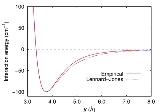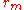xLennard-Jones potentialOverview

The Lennard-Jones potential (also referred to as the L-J potential, 6-12 potential, or 12-6 potential) is a mathematically simple model that approximates the interaction between a pair of neutral atoms or molecules. A form of the potential was first proposed in 1924 by John Lennard-Jones
John Lennard-Jones
Sir John Edward Lennard-Jones KBE, FRS was a mathematician who was a professor of theoretical physics at Bristol University, and then of theoretical science at Cambridge University...

. The most common expressions of the L-J potential arewhere ε is the depth of the potential well
Potential well
A potential well is the region surrounding a local minimum of potential energy. Energy captured in a potential well is unable to convert to another type of energy because it is captured in the local minimum of a potential well...

, σ is the finite distance at which the inter-particle potential is zero, r is the distance between the particles, andis the distance at which the potential reaches its minimum.Discussions